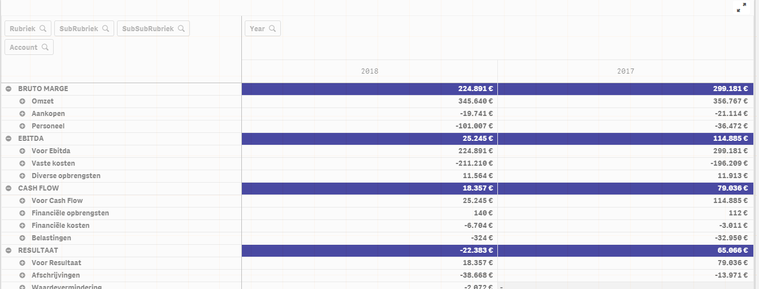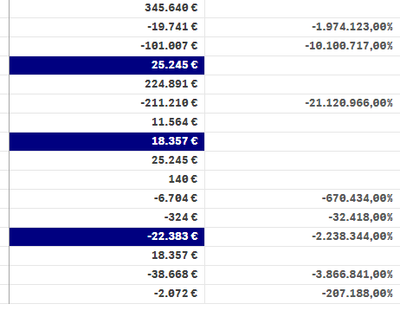# New to Qlik Sense

If you’re new to Qlik Sense, start with this Discussion Board and get up-to-speed quickly.

Announcements
Our May 2021 end-to-end product release from Data Integration to Data Analytics is out! READ DETAILS
cancel
Showing results for
Did you mean:Creator

## Dividing for percentage

Good day everyone,

I am making a profit loss statement for a client of mine,Now iWould have to get a percentage as a second measure.

This percentage should represent the number divided by the number shown next to "OMZET" =  345.640 for 2018

OMZET is the total amount of the 70 accounts. So that every measures shows how much percent it takes from the OMZET.

Any ideas how?

To achieve the above, I already had to make an excel for the parameters, wich accounts to use.

Dries

6 RepliesCreator
Author

This is what i'm trying right now:

if(sum(Debet) > sum(Credit), sum(Credit) - sum(Debet)) &' '& if(sum(Credit) > sum(Debet), sum(Credit) - sum(Debet))

/

if(SubRubriek = 'Omzet', if(sum(Debet) > sum(Credit), sum(Credit) - sum(Debet))) &' '& if(SubRubriek = 'Omzet', if(sum(Credit) > sum(Debet), sum(Credit) - sum(Debet)))Partner

Hi,

calculate

Your Expression / sum({<Rubriek = {OMZET}>} total value)

and format it to decimal in numbers

RegardsCreator
Author

Hello,

if(sum(Debet) > sum(Credit), sum(Credit) - sum(Debet)) &' '& if(sum(Credit) > sum(Debet), sum(Credit) - sum(Debet))

/

sum({<SubRubriek = {'Omzet'}>} total value )

This returns the same number as the original formule x 100Partner

2. sum (total value) is a placeholder for your correct expressionCreator
Author

Hello,

but I don't understand.

So like this?

if(sum(Debet) > sum(Credit), sum(Credit) - sum(Debet)) &' '& if(sum(Credit) > sum(Debet), sum(Credit) - sum(Debet))

/

sum({<SubRubriek = {'Omzet'}>} total if(sum(Debet) > sum(Credit), sum(Credit) - sum(Debet)) &' '& if(sum(Credit) > sum(Debet), sum(Credit) - sum(Debet)))Creator
Author

hello,

I think it has something to do with negative outcome.

The + numbers are correct,

but the - numbers show an insane percentage.